Get inspired by the success stories of our students in IIT JAM MS, ISI  MStat, CMI MSc DS.  Learn More

# ISI MStat PSA 2019 Problem 17 | Limit of a functionThis is a beautiful problem from ISI Mstat 2019 PSA problem 17 based on limit of a function . We provide sequential hints so that you can try this.

## Limit of a function

If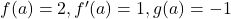and, then what is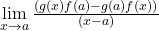?

• 5
• 3
• - 3
• -5

### Key Concepts

Algebraic manipulation

Limit form of the Derivative

ISI MStat 2019 PSA Problem 17

Introduction to real analysis Robert G. Bartle, Donald R., Sherbert.

## Try with Hints

Try to manipulate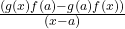so that you can use the Limit form of the Derivative . Let's give a try .=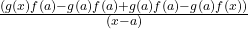=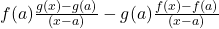.

Now calculate the limit using Limit form of the Derivative.

So, we have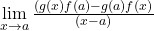=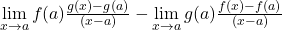=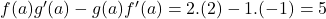.

## Subscribe to Cheenta at Youtube

This is a beautiful problem from ISI Mstat 2019 PSA problem 17 based on limit of a function . We provide sequential hints so that you can try this.

## Limit of a function

Ifand, then what is?

• 5
• 3
• - 3
• -5

### Key Concepts

Algebraic manipulation

Limit form of the Derivative

ISI MStat 2019 PSA Problem 17

Introduction to real analysis Robert G. Bartle, Donald R., Sherbert.

## Try with Hints

Try to manipulateso that you can use the Limit form of the Derivative . Let's give a try .==.

Now calculate the limit using Limit form of the Derivative.

So, we have==.

## Subscribe to Cheenta at Youtube

This site uses Akismet to reduce spam. Learn how your comment data is processed.

### Knowledge Partner